resample.h File Reference
#include <cassert>
#include <cstdlib>
#include <string>
#include <vector>
#include "matrix/matrix-lib.h"
#include "util/common-utils.h"
#include "base/kaldi-error.h"
Include dependency graph for resample.h: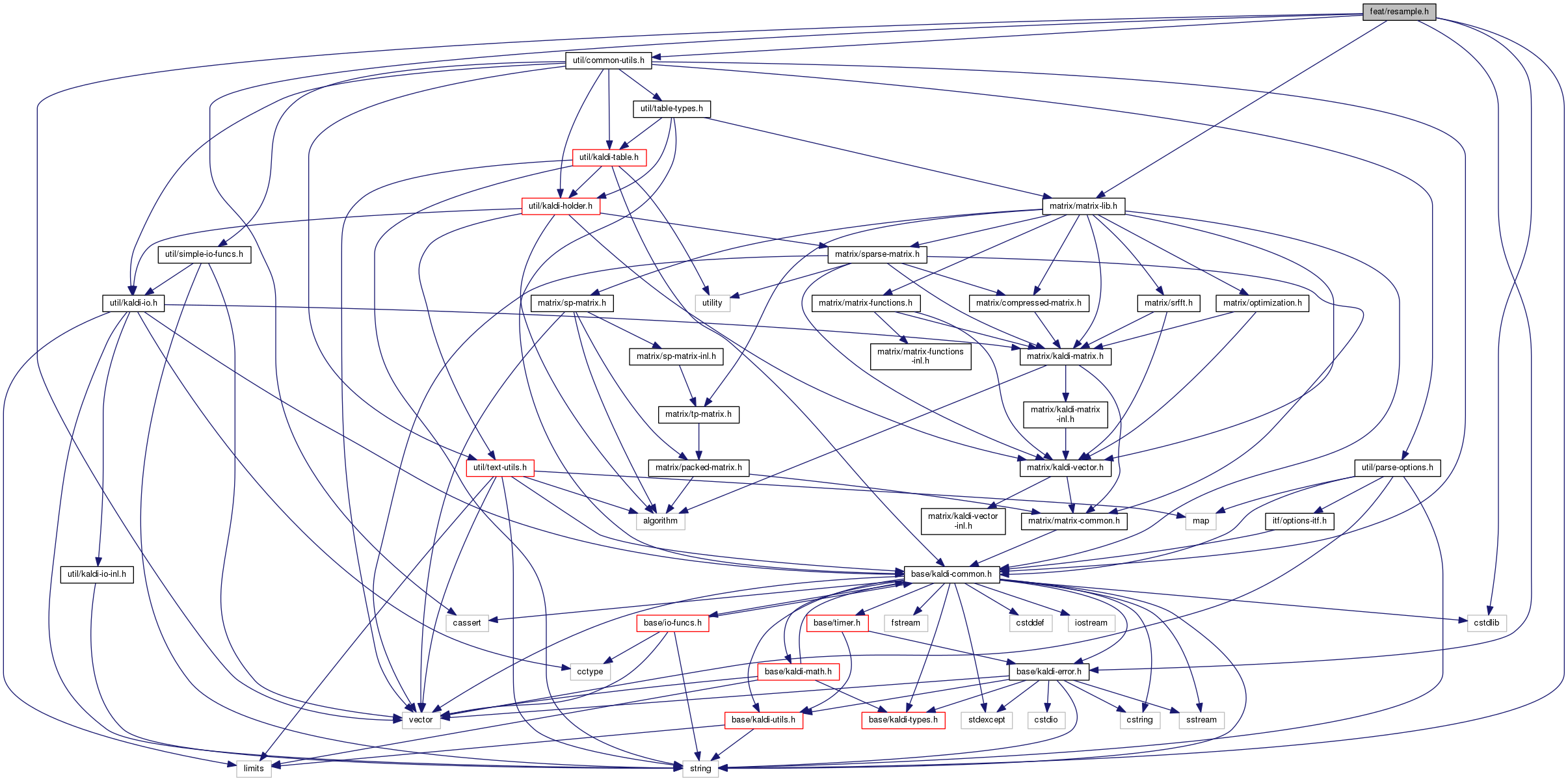This graph shows which files directly or indirectly include this file:Go to the source code of this file.

## Classes

class  ArbitraryResample
Class ArbitraryResample allows you to resample a signal (assumed zero outside the sample region, not periodic) at arbitrary specified time values, which don't have to be linearly spaced. More...

class  LinearResample
LinearResample is a special case of ArbitraryResample, where we want to resample a signal at linearly spaced intervals (this means we want to upsample or downsample the signal). More...

## Namespaces

kaldi
This code computes Goodness of Pronunciation (GOP) and extracts phone-level pronunciation feature for mispronunciations detection tasks, the reference:

## Functions

void ResampleWaveform (BaseFloat orig_freq, const VectorBase< BaseFloat > &wave, BaseFloat new_freq, Vector< BaseFloat > *new_wave)
Downsample or upsample a waveform. More...

void DownsampleWaveForm (BaseFloat orig_freq, const VectorBase< BaseFloat > &wave, BaseFloat new_freq, Vector< BaseFloat > *new_wave)
This function is deprecated. More...

## Detailed Description

]

This header contains declarations of classes for resampling signals. The normal cases of resampling a signal are upsampling and downsampling (increasing and decreasing the sample rate of a signal, respectively), although the ArbitraryResample class allows a more generic case where we want to get samples of a signal at uneven intervals (for instance, log-spaced).

The input signal is always evenly spaced, say sampled with frequency S, and we assume the original signal was band-limited to S/2 or lower. The n'th input sample x_n (with n = 0, 1, ...) is interpreted as the original signal's value at time n/S.

For resampling, it is convenient to view the input signal as a continuous function x(t) of t, where each sample x_n becomes a delta function with magnitude x_n/S, at time n/S. If we band limit this to the Nyquist frequency S/2, we can show that this is the same as the original signal that was sampled. [assuming the original signal was periodic and band limited.] In general we want to bandlimit to lower than S/2, because we don't have a perfect filter and also because if we want to resample at a lower frequency than S, we need to bandlimit to below half of that. Anyway, suppose we want to bandlimit to C, with 0 < C < S/2. The perfect rectangular filter with cutoff C is the sinc function,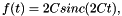where sinc is the normalized sinc function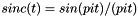, with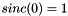. This is not a practical filter, though, because it has infinite support. At the cost of less-than-perfect rolloff, we can choose a suitable windowing function g(t), and use f(t) g(t) as the filter. For a windowing function we choose raised-cosine (Hanning) window with support on [-w/2C, w/2C], where w >= 2 is an integer chosen by the user. w = 1 means we window the sinc function out to its first zero on the left and right, w = 2 means the second zero, and so on; we normally choose w to be at least two. We call this num_zeros, not w, in the code.

Convolving the signal x(t) with this windowed filter h(t) = f(t)g(t) and evaluating the resulting signal s(t) at an arbitrary time t is easy: we have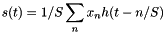. (note: the sign of t - n/S might be wrong, but it doesn't matter as the filter and window are symmetric). This is true for arbitrary values of t. What the class ArbitraryResample does is to allow you to evaluate the signal for specified values of t.

Definition in file resample.h.